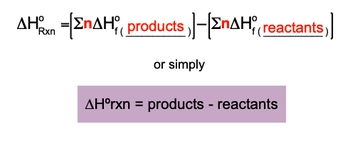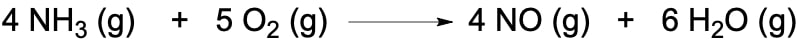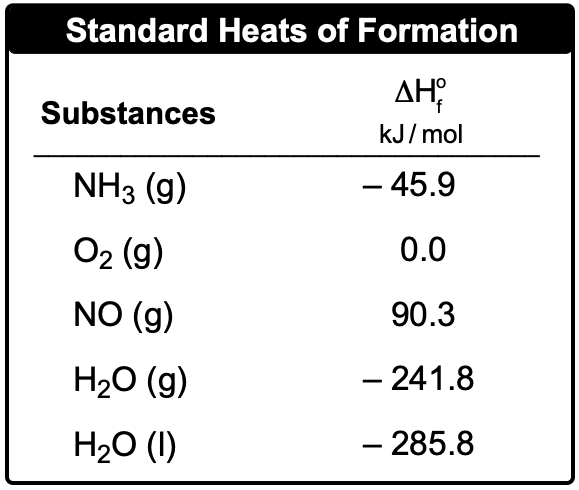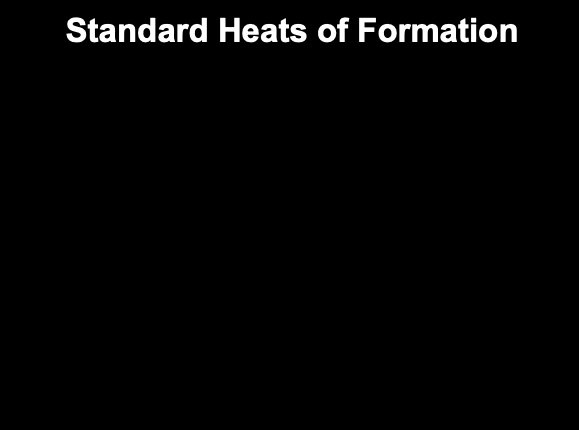## General Chemistry

Learn the toughest concepts covered in Chemistry with step-by-step video tutorials and practice problems by world-class tutors

8. Thermochemistry

# Enthalpy of Formation

The Enthalpy of Formation for an element is a key component in determining the enthalpy of reaction.

Enthalpy of Formation
1
concept

## Enthalpy of Formation2m
Play a video:
So at this point, we have identified three ways to determine the entropy, or heat of reaction, otherwise known as Delta H of reaction. Here we have constant volume Kalorama Tree, which uses a bomb calorie meter to find the entropy of reaction or heat of reaction off combustion reactions. Then we have thermal chemical equations, which uses stoy, geometry and a balanced equation to find Delta h of reaction. Then, finally, Hess's law. If you haven't seen it yet, it uses the n tal piece of partial reactions to find Delta h of reaction for the overall reaction. Now, if the first three ways air unavailable, then we use the standard and fill appeal formation for substances to find the Delta h of reaction. We're going to say, recall that element in its standard state is given an entropy of formation off zero. So here we can use our standards standard heat of reaction formula to help us find Delta h of reaction. Here it's Delta H of reaction in its standard state. That's what this little zero means here equals the summation for the centerpiece of formation of products, minus the summation of the centerpiece of formation for reactions or broken down. In its simplest way, Delta H of reaction equals products minus react. It's so here. Delta H Little Circle on top reaction. This is the standard entropy or reaction? Heats of reaction. Yeah, in killing jewels, this Sigma side just means the summation or some up, and we'll see how that works here and equals the moles of your substance. And then here we're going to say that Delta H F with the little circle equals the standard entropy, or heat of formation for substance in killing jewels over moles or sometimes jewels over moles. Depending on what units they are, it's always gonna be killed, jewels or jewels over moles. So now that we've seen this standard heat of form of reaction formula, we're going to see how it works and use it in the next video. So click on the next video. Let's take a look at example, question2
example

## Enthalpy of Formation Example 13m
Play a video:
here in this example question. It says the reaction of methane with chlorine gas is illustrated by the reaction below. Here. We need to calculate the entropy of reaction the standard entropy of reaction if the standard entropy ease off formation for methane, carbon tetrachloride and hydrochloric acid are negative. 74.87 1 negative 1 39 and negative. 92.31 killer jewels per mole, respectively. All right, so step zeros. We check to see if the chemical reaction is balanced and if not, then do the necessary steps to balance it here. We already have the equation balance. So that's great. That means we can move on to step one. Step one says starting with the products multiply the coefficients, sold the numbers and read off each substance with their entropy off formation value. Alright, So here we're going to say that we have one mole off carbon tetrachloride and four moles of HCL. We said here that carbon tetrachloride has a value associated with negative 39 kg promote and hydrochloric acid has negative 92.31 Here, the moles will cancel out and we'll have killer jewels left So this is negative. 9 to 5.24 killer jewels. Next we're going toe, go to the reactant. Also multiplied their coefficients off each substance with their rental piece of formation. Not realize here that for C l two I didn't give us an entropy formation because earlier we said that if an element is in its standard or natural state, it's entropy. Formation is equal to zero C l two. Gas is the natural form off chlorine. So it has an entropy of zero. Methane is negative, 74.87 we have one more. And here we have formals moles cancel out and what we left with killer jewels four times 00 So that doesn't matter. So this is just simply negative 74.87 killer jewels. Now that we have the amount for products and reactions, we go to step three. We take both totals and place them into the standard heat of reaction formula to determine the standard entropy of reaction. So products was negative. 9. 25.24 Killer jewels and reactions was negative. 74.87 Killer jewels Delta H Standard dealt on entropy of reaction equals products minus reactions. When we plug that in, that gives us negative 8 50.37 killer jewels as our answer. So yet this is another way that we're able to calculate the standard entropy of reaction. In this case, we did it through the use of the entire piece of formation for each of the substances within the chemical reaction.
3
Problem

The oxidation of ammonia is illustrated by the following equation:Calculate the enthalpy of reaction, ΔHRxn, based on the given standard heats of formation.4
Problem

Consider the following equation:

2 ClF3(g) + 2 NH3(g) → 1 N2(g) + 6 HF (g) + 6 Cl2(g)  ΔHrxn = –1196 kJ

Determine the standard enthalpy of formation for chlorine trifluoride, ClF3